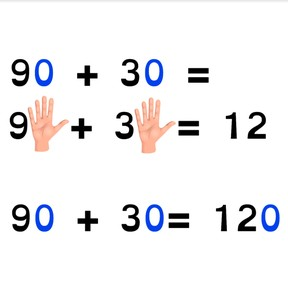Addition to 100 in tens with the zero rule

# Addition to 100 in tens with the zero rule8,000 schools use Gynzy92,000 teachers use Gynzy1,600,000 students use Gynzy

## General

Students learn to add with tens to 100 using the zero rule. They learn to solve the problem without zeros and then to use that total to help solve the actual addition problem.

2.NBT.B.5

## Relevance

If students learn to add to 100 with the zero rule, they learn to add tens faster.

## Introduction

Refresh addition problems to 10 by playing a ball game. The teacher says an addition problem (like 3+4) and tosses the ball to a student. They must say the total of the given addition problem. The student tosses the ball back to the teacher and this is repeated a few times.

## Development

Discuss that it is important to learn how to work with the zero rule because it helps you add easier and quicker. Remind students that tens are a group of ten and that they end in a 0. Ask students if they can name tens numbers. Then show the zero rule with the addition problems of 1+1 and 10+10 with the fingers and fists as well as MAB blocks to serve as visual support. This allows students to visualize the addition problem without zeros as well as with the zeros.

Check that students can add to 100 in tens with the zero rule by asking the following questions:
- Which numbers do you count when using the zero rule?
- What do you do with tens if you want to add them quickly?

## Guided practice

Together with students, practice an exercise in which the zero rule step has already been shown. Then do two exercises in which students must determine the zero rule step as well as the total answer.

## Closing

Check that students can add tens to 100 using the zero rule by asking students what to do with tens if you want to add them easily. Ask why it is useful and have them demonstrate with 30+50. Next ask students to form groups and show addition problems with their hands to 100. They must first show the zero rule step, and then the total addition problem and their partners must give the total.

## Teaching tips

For students who have difficulties with the zero rule to 100, you can practice doing a few more addition problems together. Make sure to clearly go over the steps with the students, namely that they create the zero rule step- the addition problem without zeros, and then add the zeros to determine the answer. Start with small addition problems like 20+10 and continue to problems like 30+70.

## Instruction materials

A ball

### The online teaching platform for interactive whiteboards and displays in schools

• Save time building lessons

• Manage the classroom more efficiently

• Increase student engagement